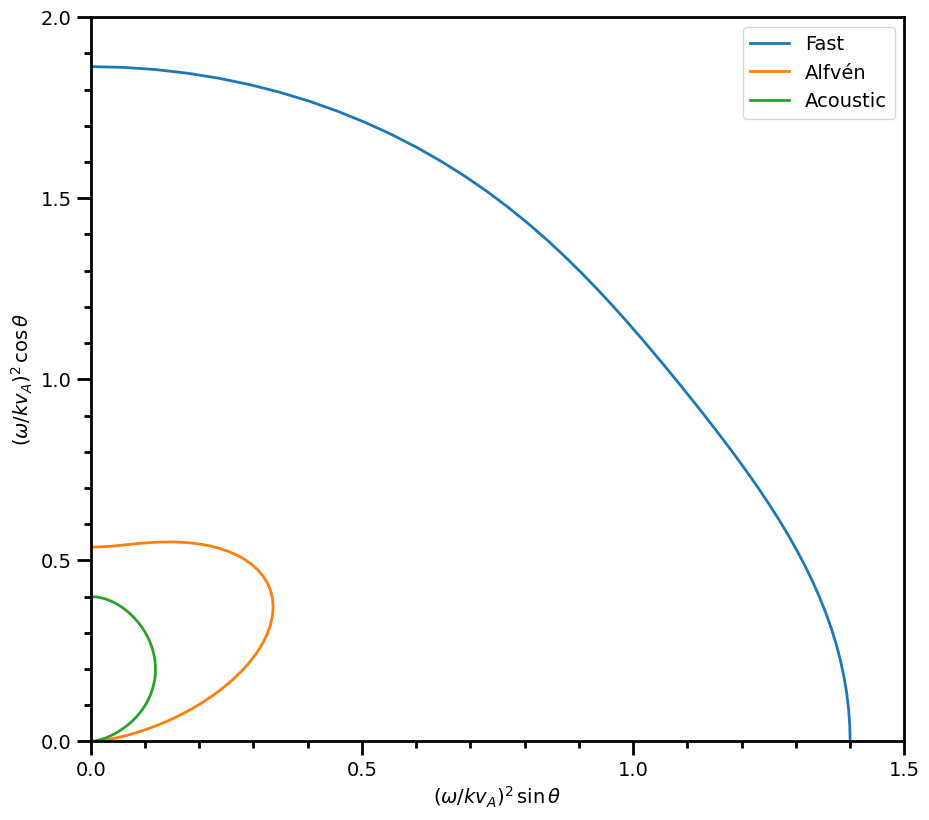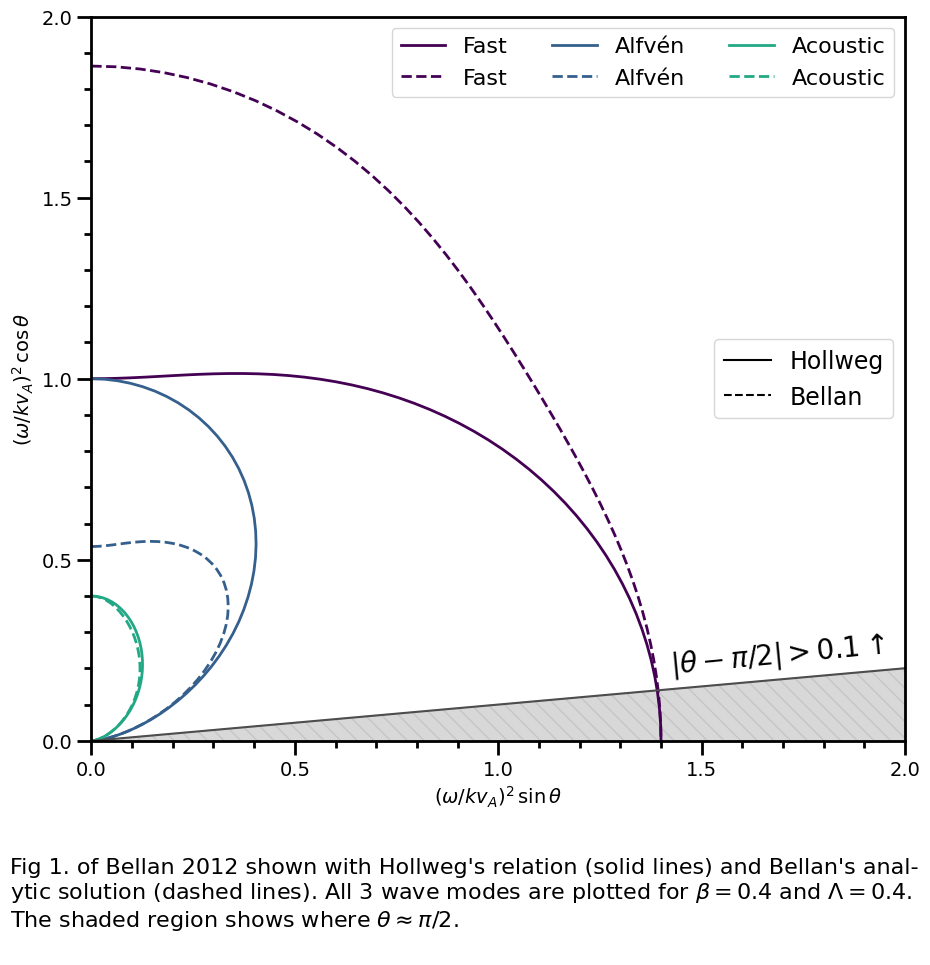# Dispersion (plasmapy.dispersion)

The dispersion subpackage contains functionality associated with plasma dispersion relations, including numerical solvers and analytical solutions.

## Sub-Packages & Modules

 analytical The analytical subpackage contains functionality associated with analytical dispersion solutions. dispersionfunction Module containing functionality focused on the plasma dispersion function $$Z(ζ)$$. numerical The numerical subpackage contains functionality associated with numerical dispersion solvers.

## Functions

 Calculate the plasma dispersion function. Calculate the derivative of the plasma dispersion function.

## Examples